Question

# A man has a simple discount note for ​\$6,200​, at an ordinary bank discount rate of...

A man has a simple discount note for ​\$6,200​, at an ordinary bank discount rate of 8.53%, for 50 days. What is the effective interest​ rate using the banker's rule?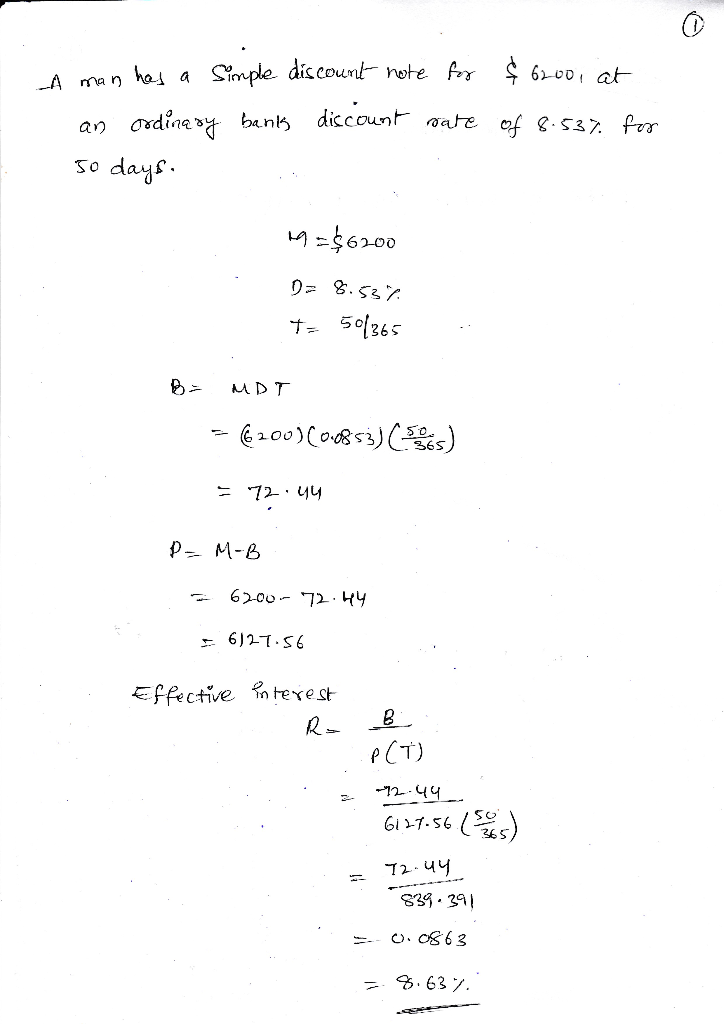A man has a simple discount note for 6,400, at an ordinary bank discount rate of 8.53%, for 60 days

#### Earn Coins

Coins can be redeemed for fabulous gifts.

Similar Homework Help Questions
• ### The face value of a simple discount note is \$4,000. The bank discount is calculated at...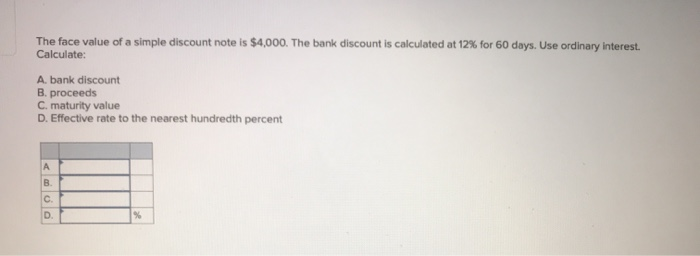The face value of a simple discount note is \$4,000. The bank discount is calculated at 12% for 60 days. Use ordinary interest. Calculate: A bank discount B. proceeds C. maturity value D. Effective rate to the nearest hundredth percent

• ### Sam Peters needs to calculate the effective interest rate of a simple discount note for \$3,600,...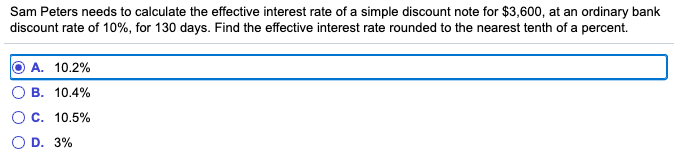Sam Peters needs to calculate the effective interest rate of a simple discount note for \$3,600, at an ordinary bank discount rate of 10%, for 130 days. Find the effective interest rate rounded to the nearest tenth of a percent. O A. 10.2% OB. 10.4% O C. 10.5% OD. 3%

• ### Bill Blank signed an \$7,540 note at Citizen's Bank. Citizen's charges a 8.2% discount rate. Assume...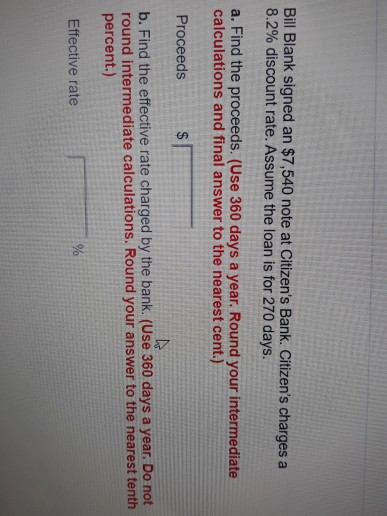Bill Blank signed an \$7,540 note at Citizen's Bank. Citizen's charges a 8.2% discount rate. Assume the loan is for 270 days. a. Find the proceeds. (Use 360 days a year. Round your intermediate calculations and final answer to the nearest cent.) Proceeds b. Find the effective rate charged by the bank. (Use 360 days a year. Do not round intermediate calculations. Round your answer to the nearest tenth percent.) Effective rate You were offered the opportunity to purchase either...

• ### Complete the following table for the simple discount notes. Use the ordinary interest method. (Use 360...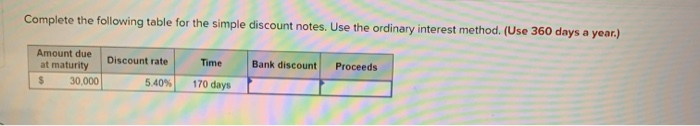Complete the following table for the simple discount notes. Use the ordinary interest method. (Use 360 days a year.) Bank discount Proceeds Amount due at maturity 30.000 Discount rate 5.40% Time 170 days

• ### Bank A charges a 12% simple interest rate and Bank B charges an 11% simple discount...

Bank A charges a 12% simple interest rate and Bank B charges an 11% simple discount rate. Show mathematically which bank has the better deal

• ### 26. When a company sells a customer's note to a bank, the discount rate is a....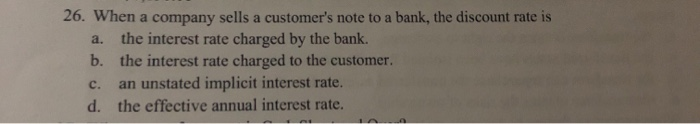26. When a company sells a customer's note to a bank, the discount rate is a. the interest rate charged by the bank. b. the interest rate charged to the customer c. an unstated implicit interest rate. d. the effective annual interest rate.

• ### the answer is 12.371% 9 A 3% discount is offered for cash payment of a \$2500...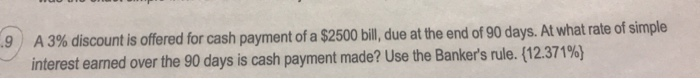the answer is 12.371% 9 A 3% discount is offered for cash payment of a \$2500 bill, due at the end of 90 days. At what rate of simple interest earned over the 90 days is cash payment made? Use the Banker's rule. (12.371%)

• ### Score: 0 of 1 pt 12 of 14 (12 complete) O Discounting Notes-1 (similar to) A...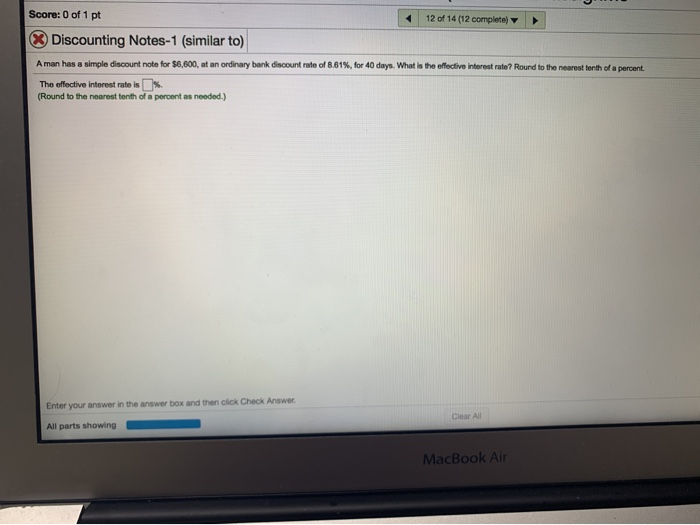Score: 0 of 1 pt 12 of 14 (12 complete) O Discounting Notes-1 (similar to) A man has a simple discount note for \$6,600, at an ordinary bank discount rate of 8.61%, for 40 days. What is the effective interest rate? Round to the nearest tenth of a percent. The offective interest rate is%. (Round to the nearest tenth of a percent as needed.) Enter your answer in the answer box and then click Check Answer. Ciear All All parts...

• ### principal: \$60,000 interest rate: 12% time (in days using ordinary interest): simple interest: \$3600 what is...

principal: \$60,000 interest rate: 12% time (in days using ordinary interest): simple interest: \$3600 what is the time in days using ordinary interest?

• ### Time Remaining: 00:5345 Submit Quiz his Question: 1 pt 5 of 8 (2 complete) This Quiz:...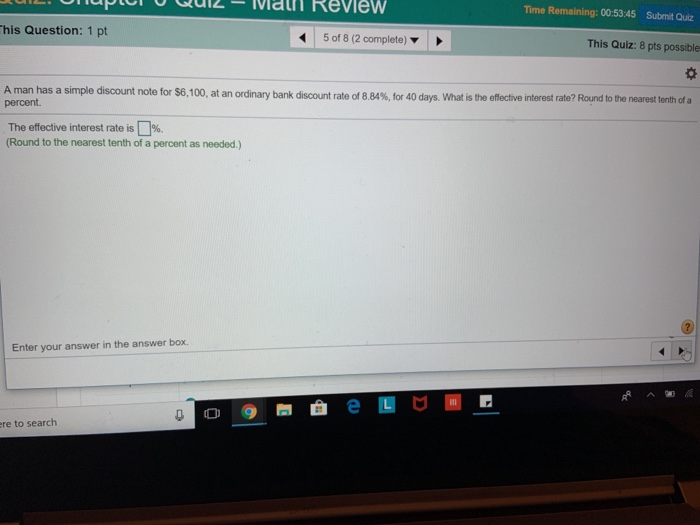Time Remaining: 00:5345 Submit Quiz his Question: 1 pt 5 of 8 (2 complete) This Quiz: 8 pts possible A man has a simple discount note for \$6,100, at an ordinary bank d percent. scount rate o 884% for 40 days. What is the effect e interest ate? Round tote earest tenth of a The effective interest rate is []%. (Round to the nearest tenth of a percent as needed.) Enter your answer in the answer box. re to search